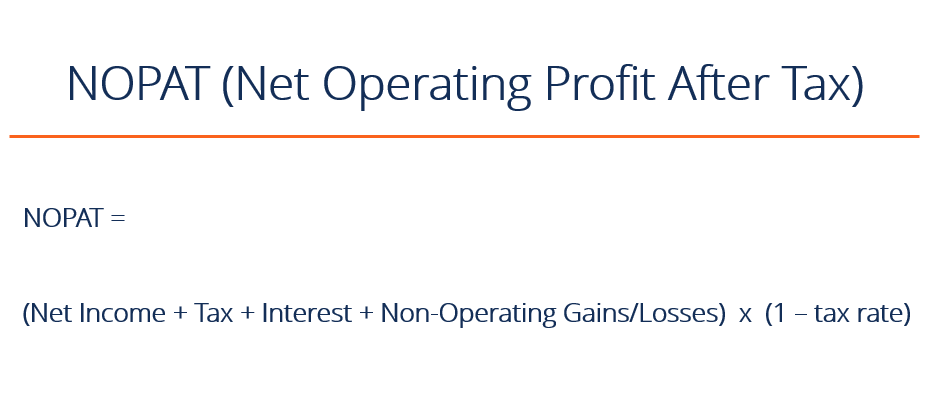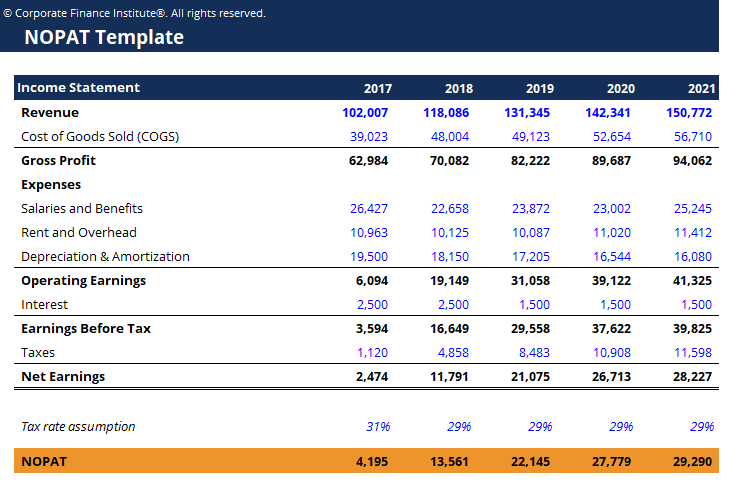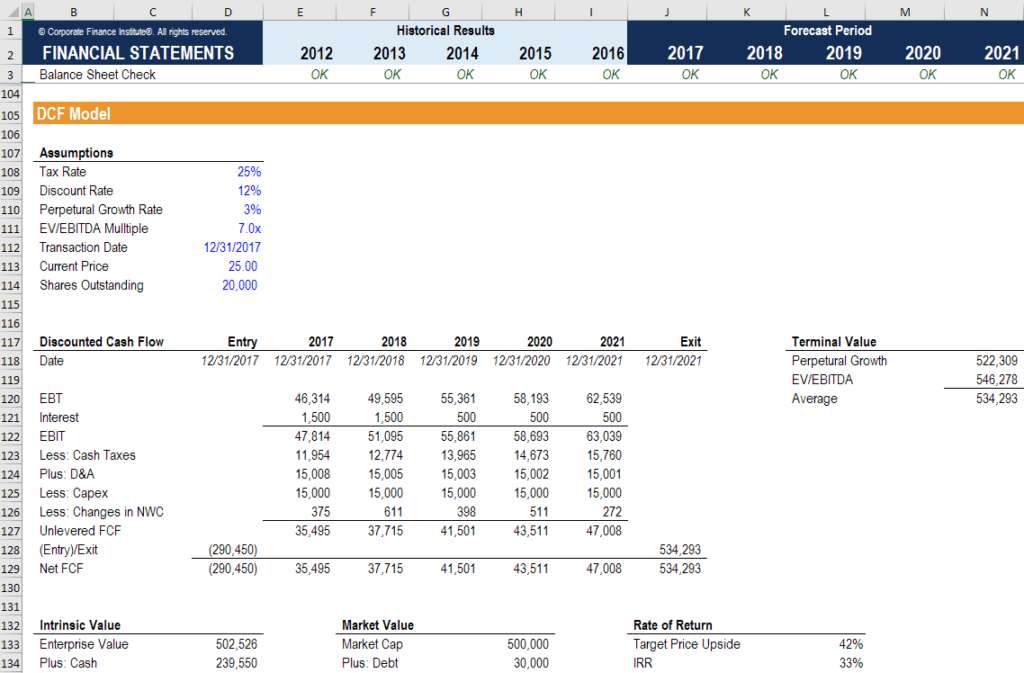# NOPAT

Net Operating Profit After Tax

## What is NOPAT?

NOPAT stands for Net Operating Profit After Tax and represents a company’s theoretical income from operations if it had no debt (no interest expense). NOPAT is used to make companies more comparable by removing the impact of their capital structure.  In this way, it’s easier to compare two companies in the same industry (i.e. one with no leverage and the other with significant leverage).

This guide will break down everything you need to know about Net Operating Profit After Tax.### NOPAT formula

The NOPAT formula is as follows:

Simple form:

Income from Operations x (1 – tax rate)

or

Long form:

[Net Income + Tax + Interest Expense + any Non-Operating Gains/Losses] x (1 – tax rate)

### NOPAT calculation example

Here is an example of how to calculate Net Operating Profit After Tax.

Please have a look at the sample income statement below, which we will use for the calculation.As you can see in the example above, in 2017 the company has Net Earnings of \$2,474 and NOPAT of \$4,195.  Let’s look at the difference between these numbers.

Operating Earnings represents the company’s profit before interest and taxes, so it shows us what the company would earn if it had not debt (no interest expense).  From there, we can calculate a new theoretical tax expense by multiplying \$6,094 by one minus the tax rate assumption of 31% (this is what the actual tax rate was in the year).

Enter your name and email in the form below and download the free template now!

### Use of NOPAT in financial modeling

In financial modeling, Net Operating Profit After Tax is used as the starting point for calculating unlevered free cash flow (a.k.a.free cash flow to the firm FCFF).

The most common approach to valuation is to calculate a firm’s enterprise value (as opposed to its equity value) so that the capital structure of the business is ignored, and only the firm’s assets are used for determining its value.

As you will see in the example below of a DCF model, the “Discounted Cash Flow” section starts with EBT, adds back interest expense, and arrives at EBIT, which is the equivalent of Operating Profit.  From there, “cash taxes” are deduced, which is based on multiplying Operating Profit (EBIT) by the tax rate.

To learn more about EBIT, EBITDA, and Cash Flow, check out CFI’s ultimate cash flow guide.The above model is taken from CFI’s financial modeling templates course.

### Difference between NOPAT and Unlevered Free Cash Flow

NOPAT does not take into account changes in net working capital accounts such as accounts receivable, accounts payable and inventory.  Additionally, it includes depreciation and amortization (a non-cash expense) and doesn’t include capital expenditures (an actual cash expense).  For these reasons, Net Operating Profit After Tax can be materially different than Free Cash Flow.

To summarize, NOPAT has the following traits:

1. Does not include changes in net working capital
2. Includes non-cash expenses such as depreciation and amortization
3. Does not include capital expenditures

To learn more, see our cash flow guide which breaks down the cash flow calculations with lost of examples.

This has been a basic guide to understanding why analysts use Net Operating Profit After Tax in their analysis and financial modeling.  With the NOPAT formula in your analyst toolkit, you can take your financial analysis to the next level.  To help advance your career, check out the resources below:

To advance your career, check out the resources below:

• Walk me through a DCF
• Financial modeling guide
• Cash flow guide
• Financial modeling and valuation courses

### Valuation Techniques

Learn the most important valuation techniques in CFI’s Business Valuation course!

Step by step instruction on how the professionals on Wall Street value a company.

Learn valuation the easy way with templates and step by step instruction!